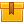# Whole Number Operations (A4301)

## Prerequisites

This lesson is designed so that no prior knowledge is required.

## Description

This lesson reviews the basic concepts of comparing, rounding, adding, subtracting, multiplying, and dividing whole numbers. It also reviews the steps for solving addition, subtraction, multiplication, and division problems. Participants learn about using estimating techniques and the calculator to solve mathematical problems. Participants also learn about a basic problem-solving strategy that can be applied to solve many mathematical problems.

## Objectives

Recognize place values. Compare values. Round whole numbers. Add whole numbers (no carrying). Add whole numbers with paper and pencil (with carrying). Add numbers with a calculator. Estimate whole numbers. Identify the steps of a problem-solving strategy. Subtract whole numbers with paper and pencil (no borrowing). Subtract whole numbers with paper and pencil (with borrowing). Subtract whole numbers with multiple zeros. Subtract whole numbers using a calculator. Estimate differences. Understand the concept of multiplication of whole numbers. Multiply whole numbers using paper and pencil (no carrying). Multiply whole numbers using paper and pencil (with carrying). Multiply whole numbers with carrying and zeros. Multiply using a calculator. Estimate product. Apply a problem-solving strategy to a multiplication problem. Recognize the concept of dividing whole numbers. Divide whole numbers using paper and pencil (with zero). Divide whole numbers using a calculator. Estimate quotient.

## ContentThis lesson reviews the basic concepts of comparing, rounding, adding,
subtracting, multiplying, and dividing whole numbers. It also reviews
the steps for solving addition, subtraction, multiplication, and
division problems. Participants learn about using estimating techniques
and the calculator to solve mathematical problems. Participants also
learn about a basic problem-solving strategy that can be applied to
solve many mathematical problems.

•Whole Number Operations

0
0 Reviews